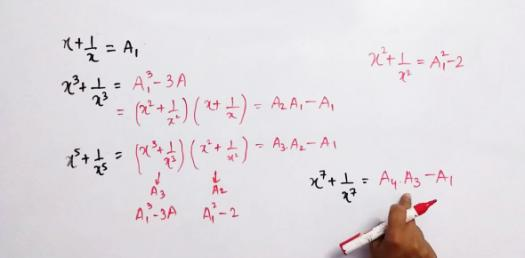# Algebra: Linear Equations Test! Trivia Questions Quiz

27 Questions | Total Attempts: 94Settings.

• 1.
5a + 12 = 3a + 20a = [Blank]
• 2.
5a - 12 = 3a + 32a=[Blank]
• 3.
10a + 23 = 5a - 12a=[Blank]
• 4.
3(a + 4) = a + 26a=[Blank]
• 5.
4(a + 12) = 2(a - 36)a=[Blank]
• 6.
3(2a - 1) = 2(2a + 12)a=[Blank]
• 7.
2(a + 4) + 3a = 3a + 32a=[Blank]
Related TopicsBack to top